# Cheese

This item is of food class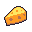You see some cheese. Eat it and see what happens.
Attributes
AMOUNT
20
FREQUENCY
5
REGEN
1
Dropped by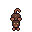mouse
Drops between 1 and 2.
Probability: 100%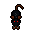caverat
Drops between 2 and 3.
Probability: 75%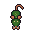venomrat
Drops between 1 and 3.
Probability: 50%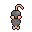zombie rat
Drops between 2 and 5.
Probability: 50%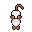razorrat
Drops between 1 and 3.
Probability: 50%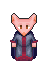ratwoman
Drops between 4 and 8.
Probability: 85%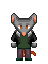ratman
Drops between 4 and 8.
Probability: 85%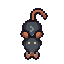giantrat
Drops between 3 and 7.
Probability: 80%